# Texas Go Math Grade 4 Lesson 14.4 Answer Key Join and Separate Angles

Refer to our Texas Go Math Grade 4 Answer Key Pdf to score good marks in the exams. Test yourself by practicing the problems from Texas Go Math Grade 4 Lesson 14.4 Answer Key Join and Separate Angles.

## Texas Go Math Grade 4 Lesson 14.4 Answer Key Join and Separate Angles

Essential Question

How can you determine the measure of an angle separated into parts?

Investigate

Materials

• construction paper
• scissors
• protractor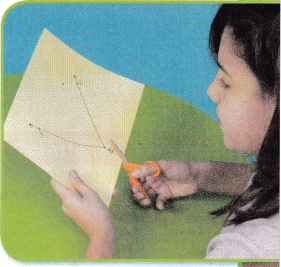A. Use construction paper. Draw an angle that measures exactly 70°. Label it ∠ABC.
The measure of angle 70° is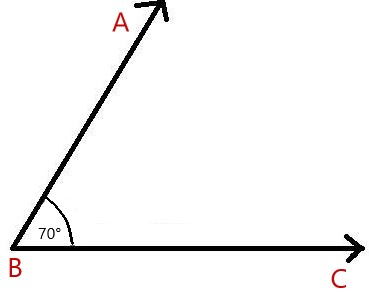B. Cut out ∠ABC.

C. Form two new angles from ∠ABCby cutting it into two parts. Begin cutting at the vertex and cut between the rays.
What figures did you form? ____________

D. Use a protractor to measure the two angles you formed.
Record the measures. ____________

E. Find the sum of the angles you formed.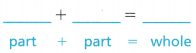Math Idea: You can think of ∠ABC as the whole and the two angles you formed as the parts of the whole.

F. Join the two angles. Compare the m∠ABC to the sum of the measures of its parts. Explain how they compare.

Make Connections

Materials
protractor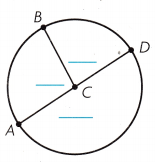You can write the measure of the angles shown in a circle as a sum.

STEP 1 Use a protractor to find the measure of each angle.
STEP 2 Label each angle with its measure.
STEP 3 Write the sum of the angle measures as an equation.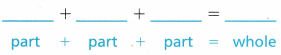Math Talk

Mathematical Processes
Describe the angles shown in the circle above using the words whole and part.

Share and Show

Add to find the measure of the angle. Write an equation to record your work.

Question 1.m∠PQT = ____________
m∠PQT = 80°.

Explanation:
The equation of m∠PQT is
m∠PQT = 43°+37°
= 80°.

Go Math Answer Key Grade 4 Join and Separate Angles Question 2.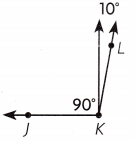m∠JKL = ______________
m∠JKL = 100°.

Explanation:
The equation of m∠JKL is
m∠JKL = 90°+10°
= 100°.

Question 3.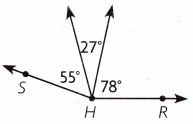m∠RHS = ______________
m∠RHS = 160°,

Explanation:
The equation of m∠RHS is
m∠RHS = 55°+27°+78°
= 160°.

Use a protractor to find the measure of each angle. Label each angle with its measure. Write the sum of the angle measures as an equation.

Question 4.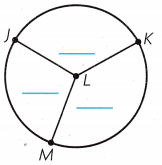m∠KLM = 160°,
m∠KLJ = 80°,
m∠LMJ = 120°.

Explanation:
By measuring each angle is
m∠KLM = 160°,
m∠KLJ = 80°,
m∠LMJ = 120°,
and the sum of the angle measures as an equation is 160°+80°+120° = 360°.

Question 5.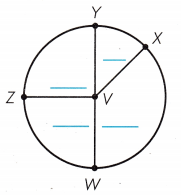m∠WVZ = 90°,
m∠YVZ = 90°,
m∠WVX = 140°,
m∠YVX = 40°.

Explanation:
By measuring each angle
m∠WVZ = 90°,
m∠YVZ = 90°,
m∠WVX = 140°,
m∠YVX = 40°,
and the sum of the angle measures as an equation is 90°+90°+140°+40° = 360°.

Unlock the ProblemH.O.T. Multi-Step Stephanie, Kay, and Shane each ate an equal-sized piece of a pizza. The measure of the angle of each piece was 45°. When the pieces were together, what is the measure of the angle they formed?
(A) 90°
(B) 135°
(C) 180°
(D) 225°
B.

Explanation:
Given that Stephanie, Kay, and Shane each ate an equal-sized piece of a pizza and the measure of the angle of each piece was 45°. So the measurement of the angle formed is 45°+45°+45° which is 135°.

a. What are you asked to find?
Here, asked to find the measurement of the angle formed.

b. What information do you need to use?
Here, we need information about the angle of each piece of pizza.

c. Justify Tell how you can use addition to solve the problem.
Here, we have used addition to know the measurement of the pizza.

d. Fill in the bubble for the correct answer choice above.

Question 7.
H.O.T. Multi-Step Reasoning ∠DRQ is a straight angle. The m∠DRB is twice the m∠QRT. What is the m∠DRB?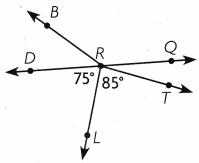(A) 40°
(B) 150°
(C) 160°
(D) 220°
A.

Explanation:
Given that m∠DRQ is a straight angle which means m∠DRQ = 180° and m∠DRL = 75°, m∠LRT = 85° the equation will be
m∠DRQ = m∠DRL+m∠LRT+m∠QRT
180° = 75°+85°+∠QRT
180° = 160°+∠QRT
∠QRT = 180°-160°
= 20°.
As m∠DRB is twice the m∠QRT, so ∠DRB is 2×20° = 40°.

Question 8.
Which equation can you use to find the m∠XZW?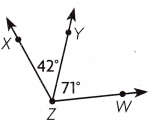(A) 71° – 42° = __________
(B) 71° + 42° = __________
(C) 71° × 42° = __________
(D) 180° – 113° = __________
B.

Explanation:
The equation will be
m∠XZW = m∠YZW+m∠XZY
=71° + 42°
= 113°.

Question 9.
Use Diagrams A sea turtle walks along a path that forms ∠ABC. What is the measure of the angle formed by the path the turtle walked?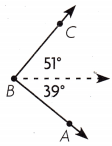(A) 51°
(B) 12°
(C) 90°
(D) 89°
C.

Explanation:
The measure of the angle formed by the path the turtle walked is
∠ABC = 51°+39°
= 90°.

4th Grade Go Math Answer Key Join and Separate Angles Lesson 14.4 Question 10.
Use Diagrams What is the m∠DEF?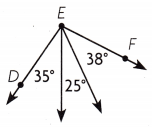(A) 90°
(B) 73°
(C) 105°
(D) 98°
D.

Explanation:
The measure of the m∠DEF is
m∠DEF = 38°+25°+35°
= 98°.

Question 11.
Multi-Step Mike used a protractor to find the measure of each angle in the circle below. What is the m∠JLK?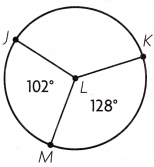(A) 360°
(B) 130°
(C) 230°
(D) 120°
C.

Explanation:
Given that m∠JLM is 102° and m∠KLM is 128° , so the measure of angle m∠JLK is 102°+128° which is 230°.

TEXAS Test Prep

Question 12.
Larry has 3 pieces of pie left. The measure of the angle of one piece is 35°. The second piece has an angle with a measure of 25°. The third piece has an angle with a measure that is half of the combined measures of the other two pieces. What is the total measure of the angle formed by all three pieces?
(A) 60°
(B) 90°
(C) 120°
(D) 180°
B.

Explanation:
Given that Larry has 3 pieces of pie left and the measure of the angle of one piece is 35° and the second piece has an angle with a measure of 25° by combing both we will get 35°+25° which is 60°. As the third piece has an angle with a measure that is half of the combined measures of the other two pieces, so the third piece is 60°÷2 which is 30°. So the total measure of the angle formed by all three pieces 60°+30° which is 90°.

### Texas Go Math Grade 4 Lesson 14.4 Homework and Practice Answer Key

Add to find the measure of the angle. Write an equation to record your work.

Question 1.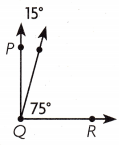m∠PQR = __________
m∠PQR = 90°.

Explanation:
The measure of the angle m∠PQR is
m∠PQR = 75°+15°
= 90°.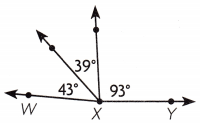m∠WXY = __________
m∠WXY = 175°.

Explanation:
The measure of the angle m∠WXY is
m∠WXY = 43°+39°+93°
= 175°.

Use a protractor to find the measure of each angle. Label each angle with its measure. Write the sum of the angle measures as an equation.

Question 3.Question 4.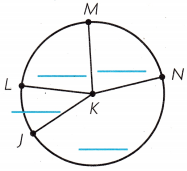Problem Solving

Question 5.
Kenny drew and cut out an angle that measured 85°. Beginning at the vertex, he cut the angle into two angles. One of new angles measures 20°. What is the measure of the other new angle?
The measure of the other new angle is 65°.

Explanation:
Given that Kenny drew and cut out an angle that measured 85° and he cut the angle into two angles, so the measure of the new angle is 20°. So the measure of the other new angle is 85°-20° which is 65°.

Question 6.
Liam and Marcy each have slices of pizza. The measure of the angle of Liam’s sLice is 40°. The measure of the angle of Marcy’s slice is 35°. When the pieces are together, what is the measure of the angle they form?
The measure of the angle they form is 75°.

Explanation:
Given that the measure of the angle of Liam’s slice is 40° and the measure of the angle of Marcy’s slice is 35°. So the pieces together are 40°+35° which is 75°.

Lesson Check

Question 7.
Which equation can you use to find the m ∠DEG?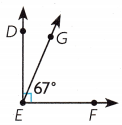(A) 67° + 90° = __________
(B) 67° × 2 = __________
(C) 180° – 90°= __________
(D) 90° – 67° = __________
D.

Explanation:
As m∠DEF is 90° m∠GEF is 67° and the equation of m∠DEF = ∠GEF+∠DEG, so ∠DEG is
m∠DEF = ∠GEF+∠DEG
90° = 67°+∠DEG
∠DEG = 90°-67°
= 23°.

Texas Go Math Grade 4 Lesson 14.4 Join and Separate Angles Question 8.
What is the m ∠KJN?(A) 100°
(B) 77°
(C) 90°
(D) 80°
D.

Explanation:
The measurement of m∠KJN is
m∠KJN = 23°+34°+43°
= 80°.

Question 9.
Hannah cut a pie into three equal parts. What is the measure of the angle of each of the parts of the pie?
(A) 30°
(B) 90°
(C) 100°
(D) 120°
D.

Explanation:
As pie is like a full circle, so the angle will be 360°. As Hannah cut a pie into three equal parts so the measure of the angle of each of the parts of the pie is 360°÷3 which is 120°.

Question 10.
Which equation can you use to find the m ∠PQR?(A) 73° – 57° = ___________
(B) 130° + 90° = ___________
(C) 73° + 57° = ___________
(D) 180° – 130° = ___________
m∠PQR = 130°.

Explanation:
The equation will be
m∠PQR = m ∠PQS+m∠SQR
= 73° + 57°
= 130°.

Multi-Step Melissa used a protractor to find the measure of each angle in the circle below. What is the m ∠WZY?(A) 218°
(B) 142°
(C) 120°
(D) 238°